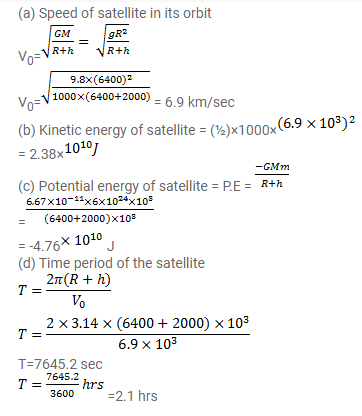# A satellite of mass 1000 kg is supposed to orbit the earth at a height of 2000 km

Question:

A satellite of mass $1000 \mathrm{~kg}$ is supposed to orbit the earth at a height of $2000 \mathrm{~km}$ above the earth's surface. Find

(a) its speed in the orbit,

(b) its kinetic energy,

(c) the potential energy of the earth-satellite system and

(d) its time period. Mass of the earth $=6 \times 1024 \mathrm{~kg}$.

Solution: